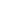# Greater Than

### This is a whole class activity.

The teacher has a set of six cards numbered 1 to 6.
They are placed face down on the teacher's desk so that the teacher can pick up one at random.

Students copy the diagram (below) showing the positioning of digits in their answer. As the teacher selects a digit the students have to choose in which cell they will write it. They cannot change their mind after they have written the digit in a cell.

#### Game 1Students are successful if the two digit number on the left is greater than the two digit number on the right.

If the student is successful he/she scores the two digit number on the right.

#### Game 2

The teacher has a set of six cards numbered 1 to 6 but this time when a number has been called out, that card goes back into the pack so it may be chosen againStudents are successful if the two digit number on the left is greater than the two digit number on the right.

If the student is successful he/she scores the two digit number on the right.

#### Game 3

Same as game 2 but this time the cards are numbered 1 to 9Students are successful if the two digit number on the left is greater than the two digit number on the right.

If the student is successful he/she scores the two digit number on the right.

#### Game 4

Same as game 2 but this time the cards are numbered 1 to 9Students are successful if the three digit number on the left is greater than the three digit number on the right.

If the student is successful he/she scores the three digit number on the right.

#### Game 5

Same as game 2 but this time the cards are numbered 1 to 9Students are successful if The two digit number on the left is greater than the two digit number in the middle which in turn is greater than the two digit number on the right.

If the student is successful he/she scores the two digit number on the right.

#### Game 6

The cards are numbered 1 to 9+-The objective is to get the largest possible value for this expression (Game 7 is to get the smallest!)

If you liked this activity you may want to try a similar idea based on simple linear equations called Nevertheless.For All: